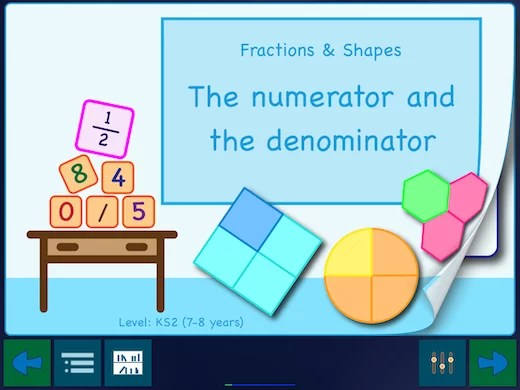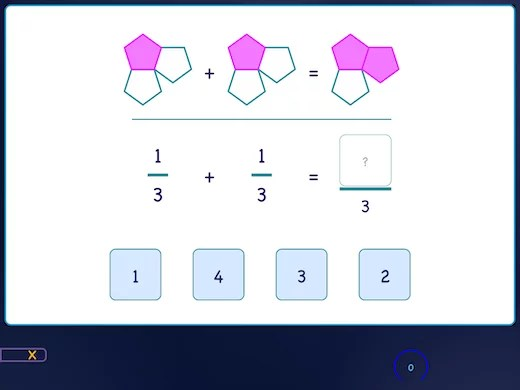Select PageFractions & Shapes app

Learn with this app the basics of calculating with fractions.

Learn to write down fractions and use them in simple additions and subtractions.

Have you had fractions during maths lessons at school and do you not understand the concept so well?
With this app, you will learn the basics of the concept of fractions, explained with the help of figures.

You first learn to recognize a fraction, determine what the denominator and numerator represent in a fraction. As soon as you understand how to write down the fraction, you will learn to calculate with fractions up to one. This is done by the app by using funny figures that represent the fractions.

The app consists of three digital exercise booklets, each with up to nine exercises with instructions.The numerator and the denominator

In these exercises, you first learn to recognize the denominator of a fraction. After you understand this correctly by doing the first three exercises, the app will move on to explain to you the concept of the numerator in a fraction. The third part of the booklet you will practice writing down the fraction together with lovely colourful (sometimes strange) figures. The booklet concludes with a test in which you can show that you understand the fractional notation.

Adding fractions together up to one

Parts can be added together. It will look strange to you, but along with the figures, you will discover this. You will see that adding fractions is just as easy as adding shapes. Finally, you can show that you can add fractions together. We do not go beyond the number 1, and you will only practise with adding parts together with the same denominators. First, you have to be able to understand the concept of adding fractions together before you start to add whole numbers or adding fraction with different denominators.Simple subtractions of fractions

When you can add fractions, then you can also subtract them from each other. However, this is done in a slightly different way. You will learn how to do this in this booklet. First, we start calculating with figures, and gradually the fractions will be added. Eventually, you learn to count without the figures. Here too we go no further than to the number 1.